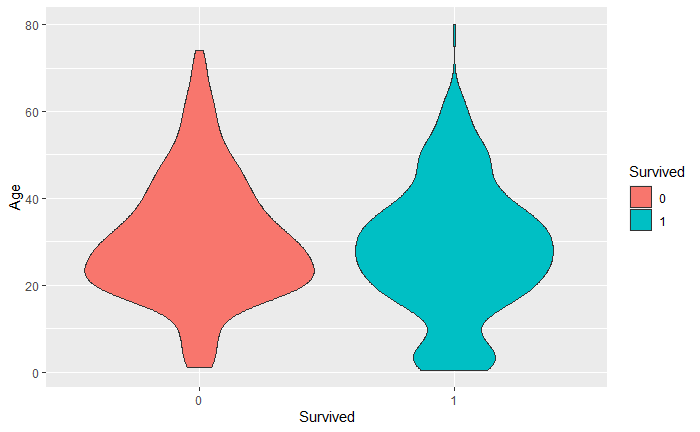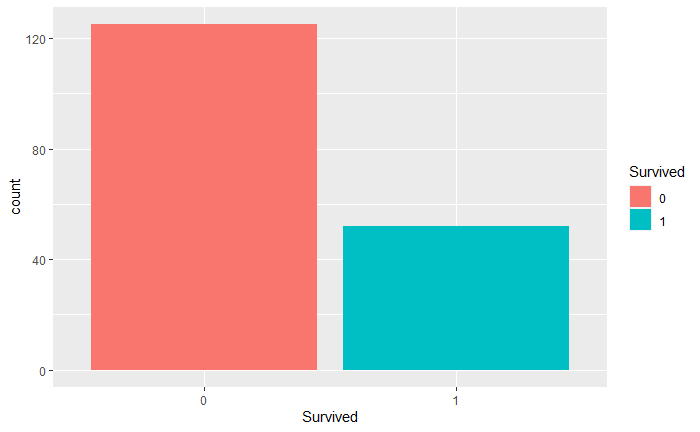Titanic Trees

Work through the kaggle Titanic competition found here with bagging, random forest, and GBM boosting.

## Libraries I might Use

``````library(tidyverse)
library(class)
library(ISLR)
library(tidyverse)
library(randomForest)
library(gbm)
library(MASS)
library(rpart)
library(caTools)``````

``````train_data = read.csv("train.csv") # Train data Is really my sample overall
test_data = read.csv("test.csv") # New predictions will be performed on this data set``````

## Encoding Factors and forcing NA for char columns

``````train_data[train_data==""] <- NA
train_data\$Sex=factor(train_data\$Sex, levels=c("male","female") ,labels = c(0,1))
train_data\$Pclass=factor(train_data\$Pclass, levels = c(1,2,3), labels = c(0,1,2))
train_data\$Embarked=factor(train_data\$Embarked, levels = c("C","Q","S"), labels = c(0,1,2))
train_data\$Survived=factor(train_data\$Survived)
train_data``````

# Exploring the data

``train_data``
``summary(train_data)``
``````  PassengerId    Survived Pclass      Name           Sex          Age            SibSp           Parch           Ticket               Fare
Min.   :  1.0   0:549    0:216   Length:891         0:577   Min.   : 0.42   Min.   :0.000   Min.   :0.0000   Length:891         Min.   :  0.00
1st Qu.:223.5   1:342    1:184   Class :character   1:314   1st Qu.:20.12   1st Qu.:0.000   1st Qu.:0.0000   Class :character   1st Qu.:  7.91
Median :446.0            2:491   Mode  :character           Median :28.00   Median :0.000   Median :0.0000   Mode  :character   Median : 14.45
Mean   :446.0                                               Mean   :29.70   Mean   :0.523   Mean   :0.3816                      Mean   : 32.20
3rd Qu.:668.5                                               3rd Qu.:38.00   3rd Qu.:1.000   3rd Qu.:0.0000                      3rd Qu.: 31.00
Max.   :891.0                                               Max.   :80.00   Max.   :8.000   Max.   :6.0000                      Max.   :512.33
NA's   :177
Cabin           Embarked
Length:891         0   :168
Class :character   1   : 77
Mode  :character   2   :644
NA's:  2

``````
1. I’m predicting Survived

2. Intuitively, I’d expect Pclass to be a significant predictor

3. Likewise, don’t women and children always go first? So age and sex should be valuable predictors.

4. SibSp represents the number of siblings/spouses aboard. Maybe important? If there are people with you they might help you escape, but you might also stay behind for them.

5. Parch is the number of parents or children abboard. If you are young I’d expect higher number of parents increases your chance, while if you are older the number of children might cause you to hold back trying to help them.

6. Information in fare is probably contained in class

7. Cabin/Ticket seem unlikely to provide much meaningful information

8. embarked is the port of embarkation. Unlikely to help much, but I’m not that confident about it.

All told our big factors are pclass, age, sex, sibsp, parch. Maybe embarked.

``````ggplot(train_data)+
geom_violin(aes(x=Survived, y = Age, fill = Survived))``````Right away we are reminded of 177 missing age values seen inthe summary.

We can also see that the didnt survive group has has a heavier weight around the age of 25.

``````ggplot(train_data[which(is.na(train_data\$Age)),])+
geom_bar(aes(x=Survived, fill = Survived))``````Most of our missing values died

``train_data[which(is.na(train_data\$Age)),]``